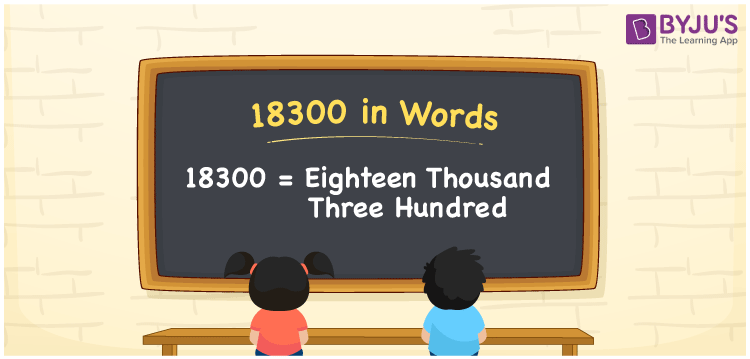# 18300 in Words

18300 in words is Eighteen thousand three hundred. The word form of a number is nothing but the name of that number. For instance, the name of the cardinal number 18300 is Eighteen thousand three hundred, which is used to convey the number to others. In this article, you will learn how to derive the word form of the number 18300 with the help of a place value chart.

 18300 in words Eighteen thousand three hundred Eighteen thousand three hundred in Numbers 18300

## 18300 in English Words

We use the English alphabet to write numbers in words and therefore, we can spell 18300 in English words as “Eighteen thousand three hundred”.## How to Write 18300 in Words?

Let’s learn how to derive 18300 in words using a place value chart. For this, we need to create a table with five columns that show the place value for five digits of the number 18300.

 Ten thousands Thousands Hundreds Tens Ones 1 8 3 0 0

Here, ones = 0, tens = 0, hundreds = 3, thousands = 8, ten thousands = 1

Let us write these digits as per their respective place values.

So, 1 × Ten thousand + 8 × Thousand + 3 × Hundred + 0 × Ten + 0 × One

= 1 × 10000 + 8 × 1000 + 3 × 100 + 0 × 10 + 0 × 1

= 10000 + 8000 + 300

= Ten Thousand + Eight thousand + three hundred

= Eighteen thousand + three hundred {since ten + eight = Eighteen}

= Eighteen thousand three hundred

18300 is a natural number that is the successor of 18299 and predecessor of 18301.

18300 in words – Eighteen thousand three hundred

Is 18300 an odd number? – No

Is 18300 an even number? – Yes

Is 18300 a prime number? – No

Is 18300 a composite number? – Yes

Is 18300 a perfect square number? – No

Is 18300 a perfect cube number? – No

## Frequently Asked Questions on 18300 in Words

Q1

### How do you write 18300 in English words?

We can write 18300 in English words as Eighteen thousand three hundred.
Q2

### Write the value of 9000 + 9300 in words.

9000 + 9300 = 18300 The value of 9000 + 9300 in words, i.e. 18300 in words is Eighteen thousand three hundred.
Q3

### What is the value of Eighteen thousand plus three hundred?

The value of Eighteen thousand plus three hundred = 18000 + 300 = 18300 = Eighteen thousand three hundred.